﻿ 基于HydroSTAR的水翼五体船耐波性优化研究
 舰船科学技术2017, Vol. 39Issue (2): 37-41PDF

1. 高新船舶与深海开发装备协同创新中心, 上海 200240;
2. 上海交通大学 船舶与海洋工程国家重点实验室, 上海 200240

Research on seakeeping performance of hydrofoil-pentamaran by HydroSTAR
KUANG Pei-qin1,2, LIU Cun-gen1,2, WANG Xue-feng1,2, ZHOU Chong-jian1,2
1. Collaborative Innovation Center for Advanced Ship and Deep-Sea Exploration, Shanghai 200240, China;
2. State Key Laboratory of Ocean Engineering, Shanghai Jiaotong University, Shanghai 200240, China
Abstract: To improve the seakeeping in small waves and no-load conditions, a new Hydrofoil-Pentamaran with four hydrofoils between main hull and accessory hulls is put forward in the paper. The seakeeping indexes of normal Petamaran and the Hydrofoil-Pentamaran with different hydrofoil angles are analyzed by HydroSTAR software. We can find from the analysis that the pitch, roll, heave will be reduced by the hydrofoil between main hull and accessory hull and the best performance angle of NACA4415 hydrofoil is about 10°.
Key words: hydrofoil     pentamaran     seakeeping
0 引 言

1 耐波性计算方程 1.1 纵摇、垂荡耦合运动方程

 ${z_R} = z - X\theta - {\rm{\zeta }}\text{，}$

 ${\zeta ^*} = {e^{ - k{T_m}}}{{\rm{\zeta }}_A}\cos \left( {kx\cos \beta - ky\sin \beta + {\omega _e}t} \right)\text{，}$

 $F{'_1} = - 2{\rm{\rho}} gb\left( {{\rm{z}} - {\rm{X\theta }} - {{\rm{\zeta }}^*}} \right)\text{，}$

 $F{'_2} = - {N_H}\left( {\dot z - X\dot \theta + V\theta - {{{\rm{\dot \zeta }}}^{\rm{*}}}} \right)\text{，}$

 $F{'_3} = - {M_H}\left( {\ddot z - X\ddot \theta + 2V\dot \theta - {{{\rm{\ddot \zeta }}}^*}} \right) + V\frac{{{\rm d}{M_H}}}{{{\rm d}X}}(\dot z - X\dot \theta + V\theta - {{\rm{\ddot \zeta }}^*})\text{，}$

 $\begin{array}{l} {F_Z} = \int\limits_L {(F{'_1} + F{'_2} + F{'_3}){\rm d}X}\text{，} \\ {M_\theta } = \int\limits_L {X(F{'_1} + F{'_2} + F{'_3}){\rm d}X} \text{。} \end{array}$

 $\begin{array}{l} \displaystyle\frac{D}{{\rm{g}}}z = {F_z}\text{，}\\ {I_{YY}}\ddot \theta = {M_\theta }\text{。} \end{array}$

1.2 横摇运动方程

 $- {I'_{xx}}\ddot \varphi - 2N\dot \varphi - Dh\varphi + Dh{a_m} = 0\text{，}$

${a_m} = {a_{{m_0}}}\sin \omega t$ ，可将平衡条件方程改写为：

 ${I'_{xx}}\ddot \varphi + 2N\dot \varphi + Dh\varphi = Dh{a_{{m_0}}}\sin \omega t\text{。}$

 $\begin{array}{l} 2\upsilon = \displaystyle\frac{{2N}}{{{{I'}_{xx}}}}\text{，}\\[8pt] \omega _\varphi ^2 = \displaystyle\frac{{Dh}}{{{{I'}_{xx}}}}\text{，} \end{array}$

 $\ddot \varphi + 2\upsilon \dot \varphi + \omega _\varphi ^2\varphi = {a_{{m_0}}}\omega _\varphi ^2\sin \omega t \text{。}$
2 耐波性计算 2.1 计算软件说明

2.2 模型参数表 1 船型参数 Tab.1 Parameter of pentamaran表 2 翼型参数 Tab.2 Parameter of hydrofoil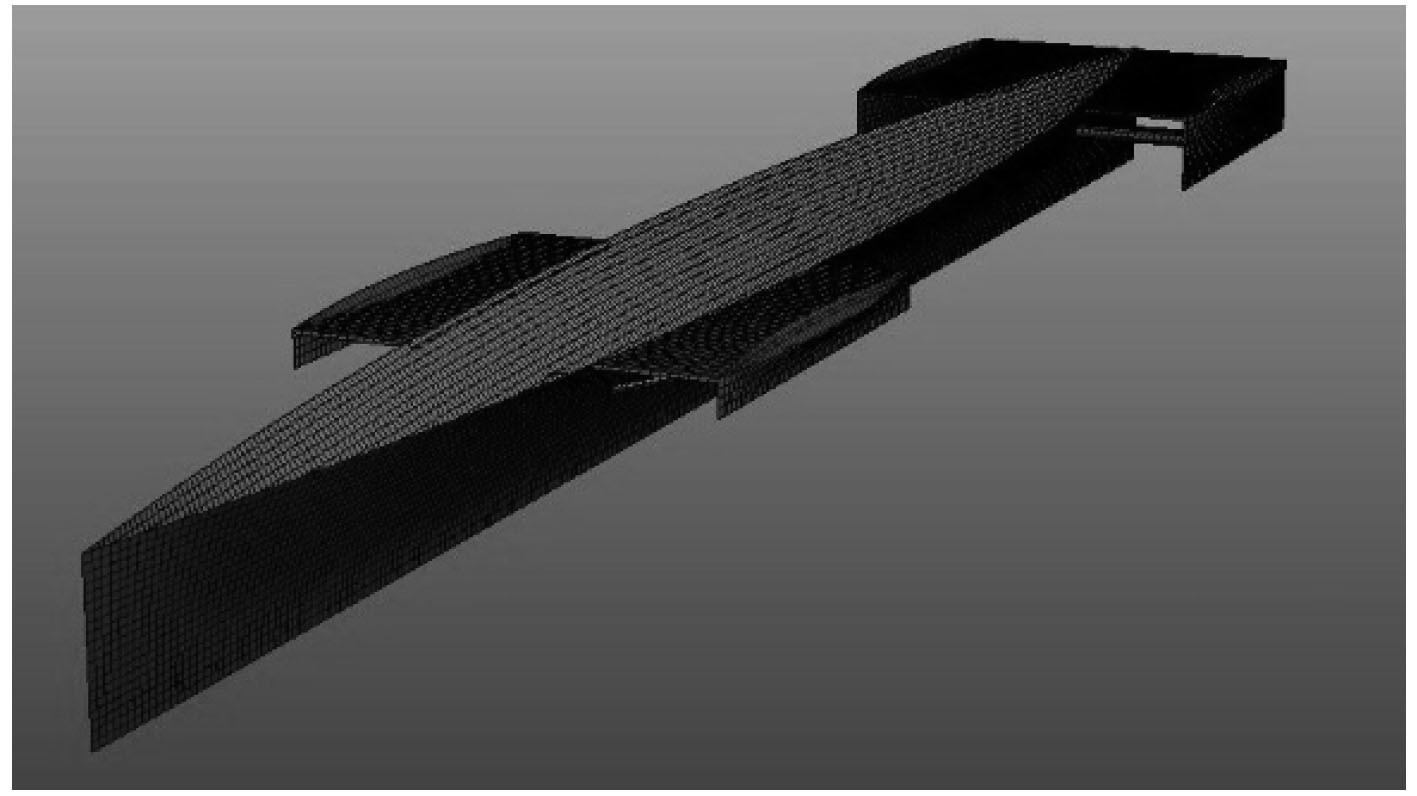图 1 水翼五体船 Fig. 1 Hydrofoil-pentamaran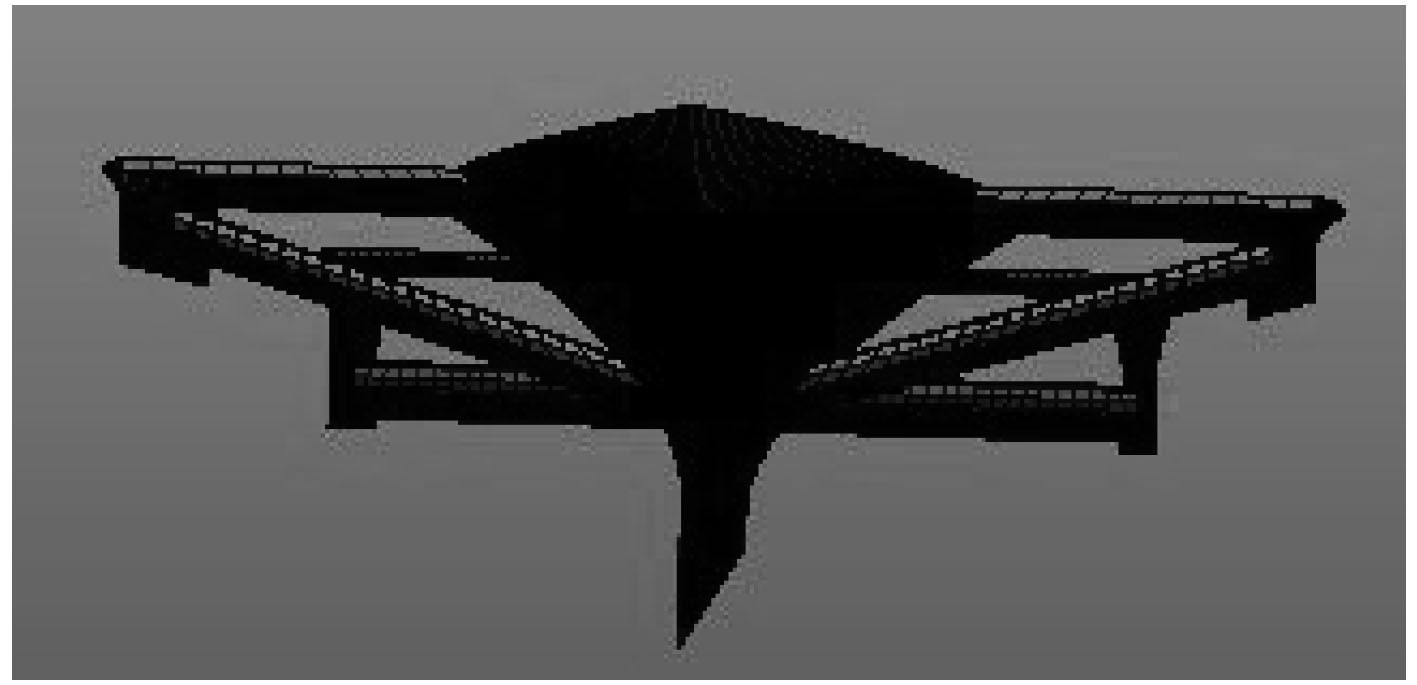图 2 水翼五体船（主视图） Fig. 2 Hydrofoil-pentamaran（Elevation）
2.3 计算设置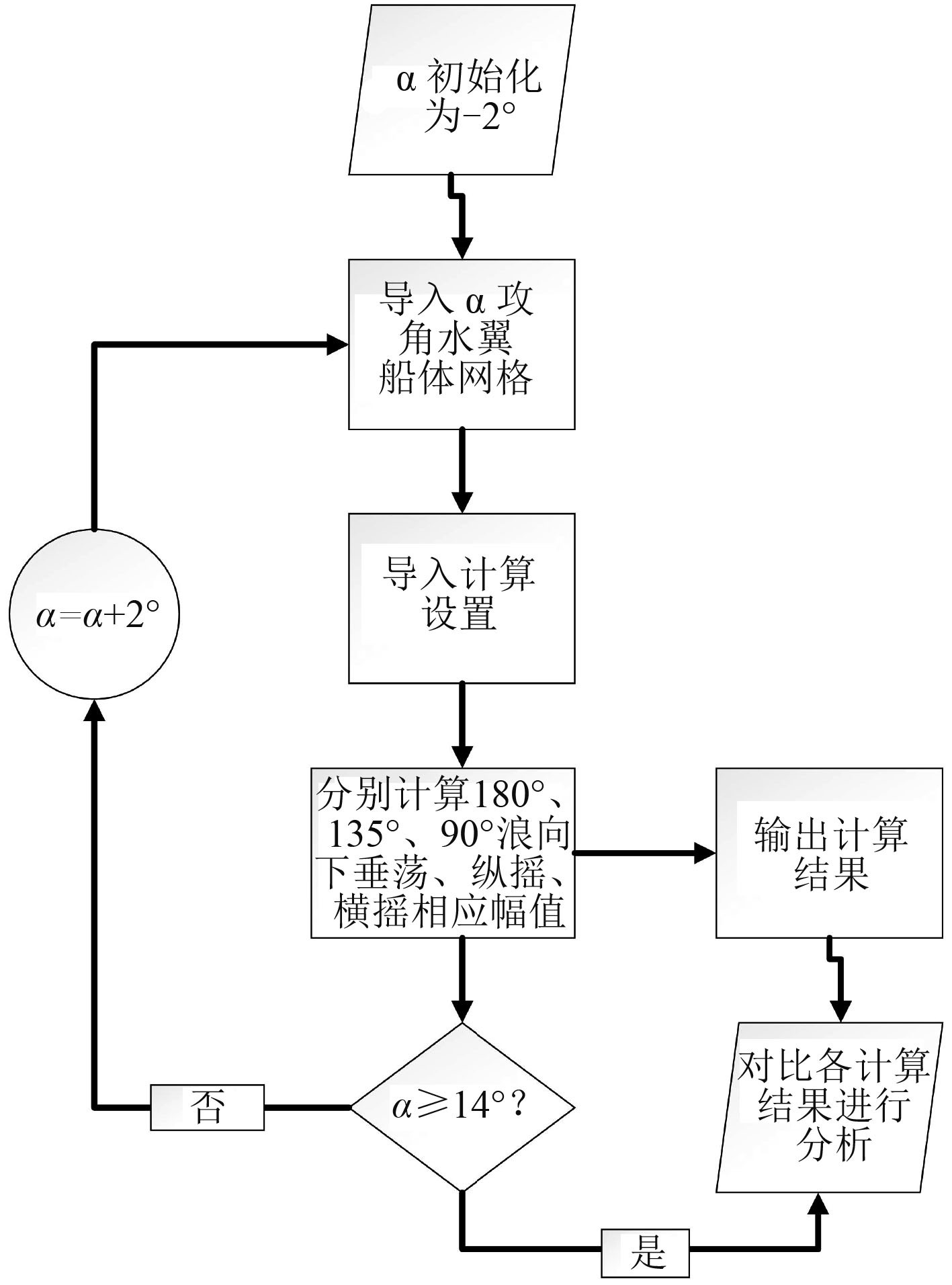图 3 分析计算流程 Fig. 3 Analysis flow chart
3 计算结果及分析

 $\begin{array}{l} {H_P} = \frac{H}{L}\text{，}\\[4pt] {P_P} = \frac{P}{L}\text{，}\\[4pt] {R_P} = \frac{R}{L}\text{。} \end{array}$

3.1 水翼五体船与五体船耐波性对比分析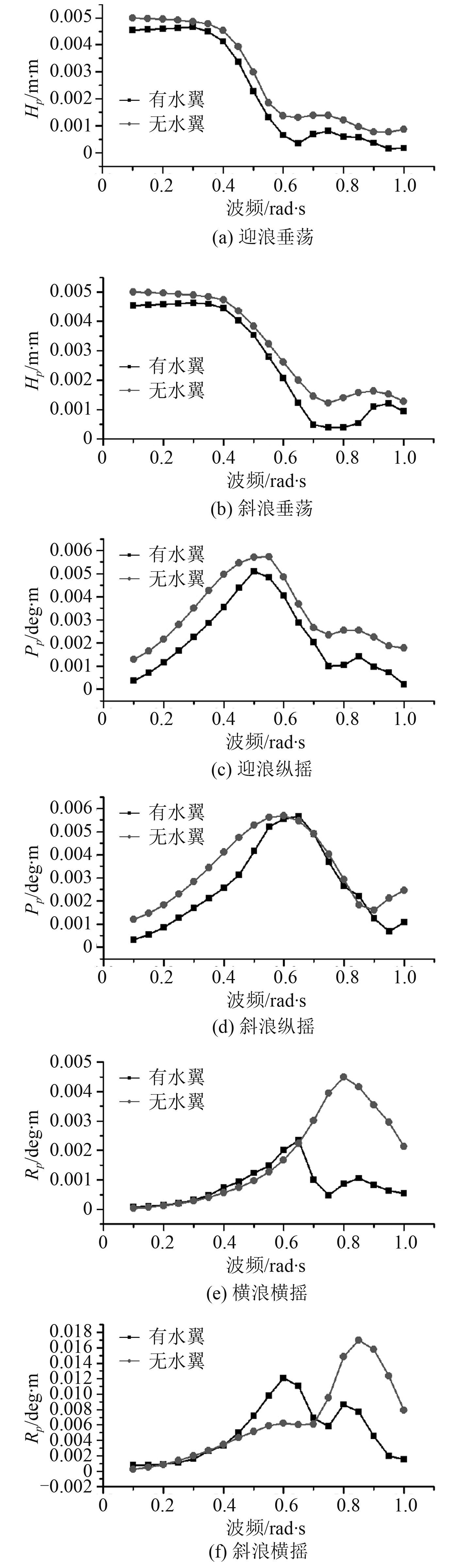图 4 水翼五体船和五体船运动响应 Fig. 4 The motion responses of hydrofoil-pentamaran and normal petamaran

3.2 水翼五体船最优攻角分析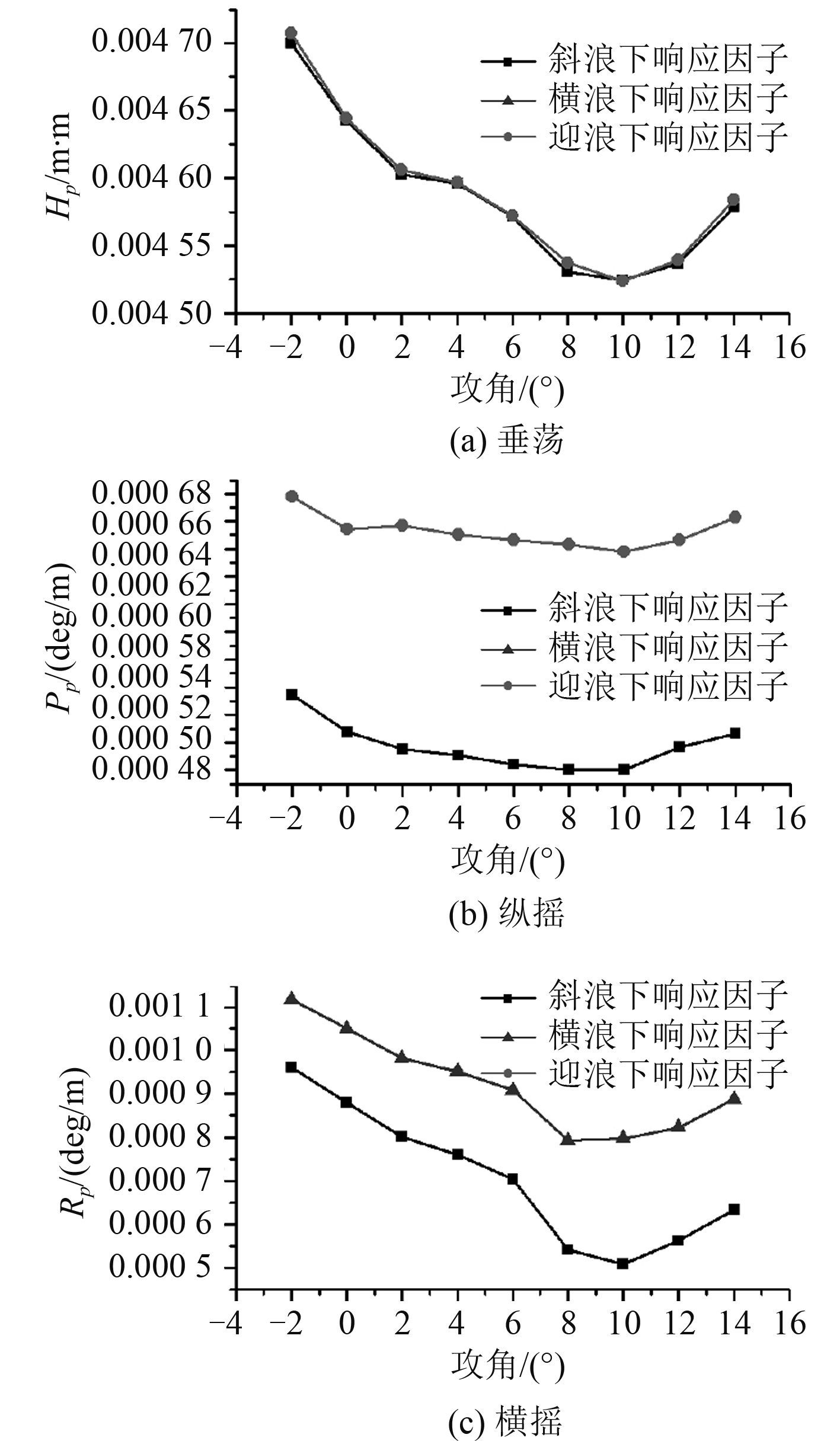图 5 各攻角水翼五体船运动响应 Fig. 5 The motion responses of hydrofoil-pentamaran with different hydrofoil angles

4 结 语

  ﻿GEE N, DUDSON E, STEIGER H. The pentamaran-A new hull concept for fast freight and car ferry applications[C]//The 13th Fast Ferry International Conference. Singapore, 1997.  DUDSON E, GEE N. Optimisation of the seakeeping and performance of a 40 knot Pentamaran container vessel[C]//Proceedings of the 6th International Conference on Fast Sea Transportation FAST2001, I. 2001.  王泽鸿, 柳存根, 周翀剑, 等. 应用五体船型降低集装箱船EEDI[J]. 船舶工程, 2014 (4): 003. WANG Ze-hong, LIU Cun-gen, ZHOU Cheng-ju, et al. Application of pentamaran to reduce EEDI of container ship[J]. Ship Engineering, 2014 (4): 003.  贺俊松, 陈震, 黄小平, 等. 五体船纵向运动预报的一种工程算法及其在前侧体位置优化中的应用[J]. 船舶力学, 2008, 3 (3): 009–387. HE Jun-song, CHEN Zhen, HUANG Xiao-ping, et al. An engineering method for predicting the longitudinal motion of pentamaran and its application in the fore sponsons' positional optimization[J]. Journal of Ship Mechanics, 2008, 3 (3): 009–387.  彭斌. 船舶节能技术综述[J]. 舰船科学技术, 2005, 27 (S1): 3–6. PENG Bin. Prospects for super energy-saving ship[J]. Ship Science and Technology, 2005, 27 (S1): 3–6.  徐敏, 张世联. 三体船/五体船的运动及波浪载荷[J]. 大连海事大学学报(自然科学版), 2011, 37 (3): 1–4. XU Min, ZHANG Shi-lian. Motions and wave loads of trimaran and pentamaran[J]. Journal of Dalian Maritime University, 2011, 37 (3): 1–4.  CALKINS D E. HYCAT:hybrid hydrofoil catamaran concept[J]. Ocean Engineering, 1984, 11 (1): 1–21. DOI: 10.1016/0029-8018(84)90021-0  CALKINS D E. Development of HYCAT[M]. 1986: 86-2381.  罗少泽, 马宁, 顾解忡, 等. 五体船阻力与耐波性协同优化方法研究[C]//2013年船舶水动力学学术会议论文集, 2013. LUO Shao-ze, MA Ning, GU Xie-chong, et al. Research on collaborative optimization for resistance and seakeeping performance of a pentamaran[C]//2013 Ship Hydrodynamics Academic Conference, 2013.  LIU S L. Numerical simulation of large amplitude ship motions and applications to ship design and safe operation[J]. 2011.  KATAM V, LEBEAU R P, JACOB J D. Experimental and computational investigation of a modified NACA 4415 in low-Re flows[C]//22nd Applied Aerodynamics Conference and Exhibit. 2004:4972.  曲立群, 汪建文, 朱德臣, 等. NACA4415翼型失速特性的二维数值研究[J]. 内蒙古工业大学学报(自然科学版), 2009 (1): 008. QU Li-qun, WANG Jian-wen, ZHU De-chen, et al. A two-dimensional numerical study on stall of NACA4415 airfoil[J]. Journal of Inner Mongolia University of Technology (Natural Science), 2009 (1): 008.  毛筱菲, 唐卫军, 熊云峰, 等. 舰船耐波性设计及其评价指标的计算[J]. 中国舰船研究, 2006, 1 (1): 41–45. MAO Xiao-fei, TANG Wei-jun, XIONG Yun-feng, et al. Seakeeping design and calculation of the ship performance index[J]. Chinese Journal of Ship Research, 2006, 1 (1): 41–45.